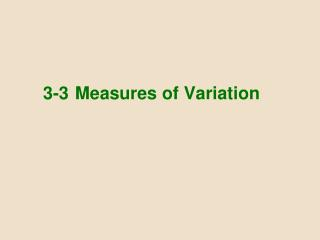Download Presentation3-3 Measures of Variation3-3 Measures of Variation - PowerPoint PPT Presentation

Download Presentation3-3 Measures of Variation
An Image/Link below is provided (as is) to download presentation

Download Policy: Content on the Website is provided to you AS IS for your information and personal use and may not be sold / licensed / shared on other websites without getting consent from its author. While downloading, if for some reason you are not able to download a presentation, the publisher may have deleted the file from their server.

- - - - - - - - - - - - - - - - - - - - - - - - - - - E N D - - - - - - - - - - - - - - - - - - - - - - - - - - -
Presentation Transcript

1. 3-3 Measures of Variation

2. Definition The range of a set of data values is the difference between the maximum data value and the minimum data value. Range = (maximum value) – (minimum value) It is very sensitive to extreme values; therefore, it is not as useful as other measures of variation.

3. Definition The standard deviation of a set of sample values, denoted by s, is a measure of how much data values deviate away from the mean.

4. Sample Standard Deviation Formula

5. Standard Deviation – Important Properties • The standard deviation is a measure of variation of all values from the mean. • The value of the standard deviation s is usually positive (it is never negative). • The value of the standard deviation s can increase dramatically with the inclusion of one or more outliers (data values far away from all others). • The units of the standard deviation s are the same as the units of the original data values.

6. Example Use the formula to find the standard deviation of these numbers of chocolate chips: 22, 22, 26, 24

7. Example

8. Range Rule of Thumb for Understanding Standard Deviation It is based on the principle that for many data sets, the vast majority (such as 95%) of sample values lie within two standard deviations of the mean.

9. = Minimum “usual” value (mean) – 2  (standard deviation) = Maximum “usual” value (mean) + 2  (standard deviation) Range Rule of Thumb for Interpreting a Known Value of the Standard Deviation Informally define usual values in a data set to be those that are typical and not too extreme. Find rough estimates of the minimum and maximum “usual” sample values as follows:

10. Range Rule of Thumb for Estimating a Value of theStandard Deviation s To roughly estimate the standard deviation from a collection of known sample data use where range = (maximum value) – (minimum value)

11. Example Using the 40 chocolate chip counts for the Chips Ahoy cookies, the mean is 24.0 chips and the standard deviation is 2.6 chips. Use the range rule of thumb to find the minimum and maximum “usual” numbers of chips. Would a cookie with 30 chocolate chips be “unusual”?

12. Example *Because 30 falls above the maximum “usual” value, we can consider it to be a cookie with an unusually high number of chips.

13. Comparing Variation inDifferent Samples It’s a good practice to compare two sample standard deviations only when the sample means are approximately the same. When comparing variation in samples with very different means, it is better to use the coefficient of variation, which is defined later in this section.

14. Population Standard Deviation This formula is similar to the previous formula, but the population mean and population size are used.

15. Variance • The variance of a set of values is a measure of variation equal to the square of the standard deviation. • Sample variance: s2 - Square of the sample standard deviation s • Population variance: σ2 - Square of the population standard deviation σ

16. Variance - Notation s=sample standard deviation s2= sample variance =population standard deviation =population variance

17. Unbiased Estimator The sample variance s2 is an unbiased estimator of the population variance , which means values of s2 tend to target the value of instead of systematically tending to overestimate or underestimate .

18. Rationale for using (n – 1) versus n There are only (n – 1) independent values. With a given mean, only (n – 1) values can be freely assigned any number before the last value is determined. Dividing by (n – 1) yields better results than dividing by n. It causes s2 to target whereas division by n causes s2 to underestimate .

19. Empirical (or 68-95-99.7) Rule For data sets having a distribution that is approximately bell shaped, the following properties apply: • About 68% of all values fall within 1 standard deviation of the mean. • About 95% of all values fall within 2 standard deviations of the mean. • About 99.7% of all values fall within 3 standard deviations of the mean.

20. The Empirical Rule

21. Chebyshev’s Theorem The proportion (or fraction) of any set of data lying within K standard deviations of the mean is always at least 1–1/K2, where K is any positive number greater than 1. • For K = 2, at least 3/4 (or 75%) of all values lie within 2 standard deviations of the mean. • For K = 3, at least 8/9 (or 89%) of all values lie within 3 standard deviations of the mean.

22. Example • IQ scores have a mean of 100 and a standard deviation of 15. What can we conclude from Chebyshev’s theorem? • At least 75% of IQ scores are within 2 standard deviations of 100, or between 70 and 130. • At least 88.9% of IQ scores are within 3 standard deviations of 100, or between 55 and 145.